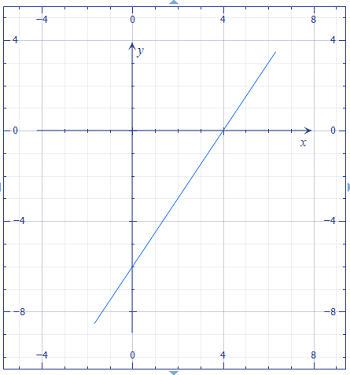# Given the linear function 6x - 4y = 24. Find a) the slope. b) the x- and y-intercept. c) Graph...

## Question:

Given the linear function 6x - 4y = 24. Find

a) the slope.

b) the x- and y-intercept.

c) Graph the function.

## Linear Equations:

We typically come across linear equations, i.e. those equations that describe lines, in one of three forms. The first is standard, which was actually standard a few hundred years ago, but is really anything but anymore. Standard form generally looks like {eq}ax + by = c {/eq} (our equation here is in standard form). The second is slope-intercept form: {eq}y = mx +b {/eq}. This really is the new standard; with it we can read the slope {eq}m {/eq} and the {eq}y {/eq}-intercept {eq}b {/eq} straight from the equation. The last is point-slope form: {eq}y - y_0 = m(x-x_0) {/eq}, which we include here only for completeness (we will not be using it in this solution).

Part A

We can get the equation in slope-intercept form, then read the slope straight from the equation. We have

{eq}\begin{align*} 6x - 4y &= 24 \\ -4y &= -6x +24 \\ y &= \frac32 x-6 \end{align*} {/eq}

so the slope is

{eq}\begin{align*} m = \frac32 \end{align*} {/eq}

Part B

We read the {eq}y {/eq}-intercept straight from the slope-intercept form of the line above. It is

{eq}\begin{align*} y = -6 \end{align*} {/eq}

To find the {eq}x {/eq}-intercept (more commonly called the zeroes), we set {eq}y = 0 {/eq} and solve for {eq}x {/eq}.

{eq}\begin{align*} \frac32 x-6 &= 0 \\ \frac32 x &= 6 \\ x &= 4 \end{align*} {/eq}

Part C

A plot is below.